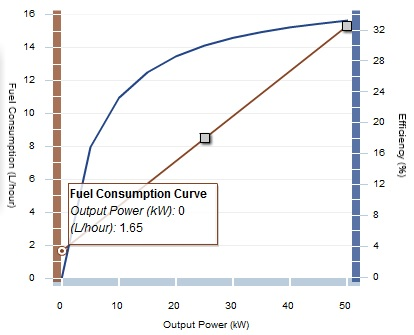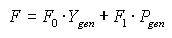# HOMER Pro 3.11

 Navigation: Glossary Generator Fuel Curve Intercept CoefficientType: Input Variable Units: fuel units/hr/kWrated Symbol: F0

The generator fuel curve intercept coefficient is the no-load fuel consumption of the generator divided by its rated capacity. If you plot a straight line of fuel consumption versus the power output of the generator, the y-intercept of that line divided by the generator size is equal to the fuel curve intercept coefficient.

For example, if a 50-kW generator consumes 8.48 L/hr at 25 kW output, and 15.3 L/hr at rated output, the slope of the fuel curve is (15.3-8.48)/(50-25) = 0.273 L/hr/kWoutput. So the y-intercept is 8.48 - (0.273*25) = 1.655 L/hr. Dividing by 50 kW (the size of the generator) gives the fuel curve intercept coefficient of 0.033 L/hr/kWrated. This fuel curve is shown below.The Fuel Curve Calculator helps calculate the fuel curve slope and intercept coefficient.

If the generator is running in a particular time step, HOMER calculates the fuel consumption rate for that time step using the following equation:where: F = fuel consumption rate [L/hr] F0 = generator fuel curve intercept coefficient [L/hr/kWrated] F1 = generator fuel curve slope [L/hr/kWoutput] Ygen = rated capacity of the generator [kW] Pgen = output of the generator in this time step [kW]

If the generator is not running in a particular time step, the fuel consumption for that time step is zero.

See also

Generator Fuel Curve Slope